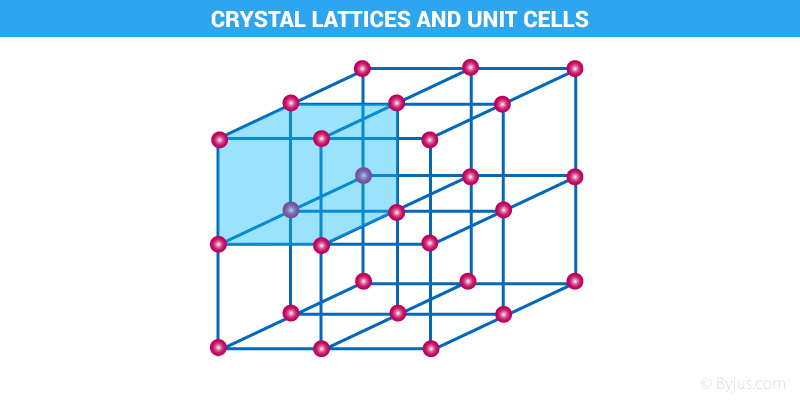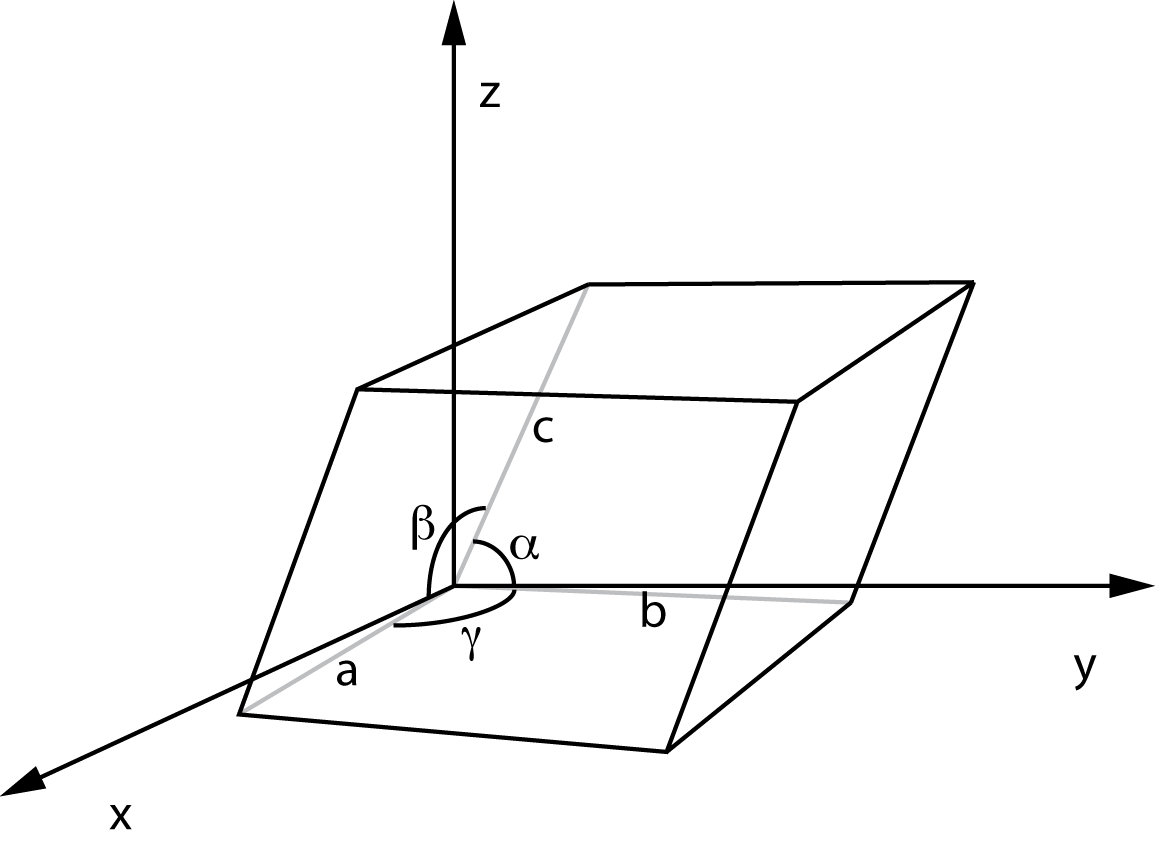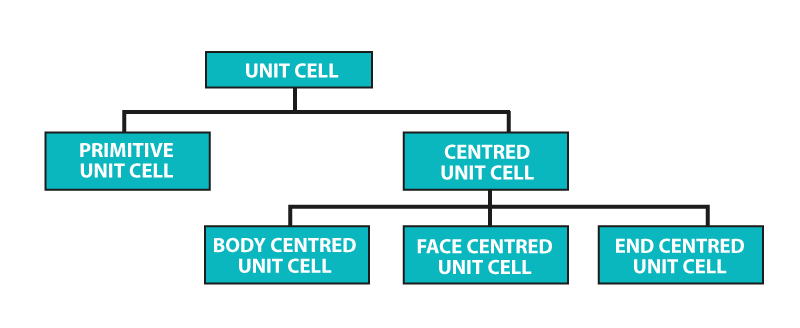# Crystal Lattices and Unit Cells

The ‘crystal lattice’ is the pattern formed by the points and used to represent the positions of these repeating structural elements. The periodic structure of an ideal crystal is most easily described by a lattice. The crystal lattice is the array of points at the corners of all the unit cells in the crystal structure.

## What is Crystal Lattice?

The crystal lattice is the symmetrical three-dimensional structural arrangements of atoms, ions or molecules (constituent particle) inside a crystalline solid as points. It can be defined as the geometrical arrangement of the atoms, ions or molecules of the crystalline solid as points in space.### Characteristics of Crystal Lattice

• In a crystal lattice, each atom, molecule or ions (constituent particle) is represented by a single point.
• These points are called lattice site or lattice point.
• Lattice sites or points are together joined by a straight line in a crystal lattice.
• When we connect these straight lines we can get a three-dimensional view of the structure. This 3D arrangement is called Crystal Lattice also known as Bravais Lattices.

## Unit Cell

Unit Cell is the smallest part (portion) of a crystal lattice. It is the simplest repeating unit in a crystal structure. The entire lattice is generated by the repetition of the unit cell in different directions.

### 1. Parameters of a Unit CellUnit Cell Parameters

There are six parameters of a unit cell. These are the 3 edges which are a, b, c and the angles between the edges which are α, β, γ. The edges of a unit cell may be or may not be perpendicular to each other.

### 2. Types of Unit CellTypes of Unit Cell

#### 1. Primitive Unit Cells

When the constituent particles occupy only the corner positions, it is known as Primitive Unit Cells.

#### 2. Centred Unit Cells

When the constituent particles occupy other positions in addition to those at corners, it is known as Centred Unit Cell. There are 3 types of Centred Unit Cells:

• Body Centred: When the constituent particle at the centre of the body, it is known as Body Centred Unit cell.
• Face Centred: When the constituent particle present at the centre of each face, it is known as Face Centred Unit cell.
• End Centred: When the constituent particle present at the centre of two opposite faces, it is known as an End Centred Unit cell.

## Recommended Videos### What is a lattice structure?

A lattice is an ordered set of points that define the structure of a crystal-forming particle. The lattice points identify the unit cell of a crystal. All the particles (yellow) are the same in the drawn structure.

### What is the lattice point in crystals?

The points in a crystal lattice in the model crystal represent the positions of structural units (atoms, molecules or ions). Every lattice point in the crystal has the same surroundings as the actual crystal structural units.

### What is a lattice point in a unit cell?

Unit cell corner is described by a lattice point at which the crystal contains an atom, ion, or molecule. The cubic unit cell centred on the body is the simplest repeating unit in a cubic structure centred on the body. Once again, the eight corners of the unit cell contain eight similar particles.

### How crystal lattice is formed?

If one mole of an ionic compound is formed, it is the energy produced. That ensures that when the compound’s individual ions come together to form the crystal lattice, they need less energy to remain together so that they release it and the energy released is called the energy of the lattice.

### What causes a crystal lattice?

The ionic crystal consists of electrostatic attraction linking ions together. The arrangement of ions is called a crystal lattice in a regular, geometric structure. The alkali halides are examples of such crystals, including: potassium fluoride (KF)